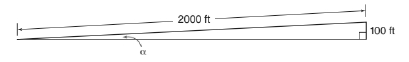Chapter 11.1, Problem 30E### Elementary Geometry for College St...

6th Edition
Daniel C. Alexander + 1 other
ISBN: 9781285195698

#### Solutions

Chapter
Section### Elementary Geometry for College St...

6th Edition
Daniel C. Alexander + 1 other
ISBN: 9781285195698
Textbook Problem
1 views

# In Exercises 27 to 34, use the drawings, where provided, to solve each problem. Angle measures should be given to the nearest degree; distances should be given to the nearest tenth of a unit.Over a 2000-ft span of highway through a hillside, there is a 100-ft rise in the roadway. What is the measure of the angle formed by the road and the horizontal?To determine

To find:

The angle formed by the road and the horizontal.

Explanation

In a right angle triangle,

1) The side PQ, which is opposite to the right angle PRQ is called the hypotenuse.

(The hypotenuse is the longest side of the right triangle.)

2) The side RQ is called the adjacent side of angle θ.

3) The side PR is called the opposite side of angle θ.

Hence, the sine ratio for an acute angle is oppositehypotenuse

Given:

From, the given diagram,

Opposite side = 100 ft and hypotenuse = 2000 ft

Let α be the angle formed by the road and the horizontal

### Still sussing out bartleby?

Check out a sample textbook solution.

See a sample solution

#### The Solution to Your Study Problems

Bartleby provides explanations to thousands of textbook problems written by our experts, many with advanced degrees!

Get Started

#### In Exercises 73-80, find the indicated limits, if they exist. 75. limx3x3+x2+1x3+1

Applied Calculus for the Managerial, Life, and Social Sciences: A Brief Approach

#### Subtract: 4000484

Elementary Technical Mathematics

#### For f(x)=7+5x2x2, find and simplify f(x+h)f(x)h

Mathematical Applications for the Management, Life, and Social Sciences

#### The fourth term of {an} defined by a1 = 3, and for n = 1, 2, 3, … is:

Study Guide for Stewart's Single Variable Calculus: Early Transcendentals, 8th